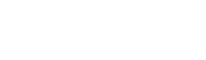# 【Python】NetworkX which draws networks in a diagram

スポンサーリンク

Hello, this is Yuki (@engineerblog_Yu), a student engineer.

Those who have finished learning the basics of Python.

Those who need to create network diagrams for college or work.

Those who want to create graph theory diagrams with programming.

Those who are fascinated by graphs and have a passion for science.

スポンサーリンク

## What is NetworkX?

NetworkX is a Python library package used for graph theory and network theory.

It can be used in conjunction with Matplotlib and PYGraphviz to visualize as images.

## How to use NetworkX

pip install networkx
import matplotlib.pyplot as plt
import networkx as nx

we initialize the graph.

This time, we want to create an undirected graph that does not take orientation into account.

G = nx.Graph()

If you want to create a valid graph, you can type in the code here and the following is the same as for an undirected graph.

G = nx.DiGraph()

G.add_node(1)

## Image display

import matplotlib.pyplot as plt
import networkx as nx

G = nx.Graph()

nx.draw(G)
plt.show()

You will see one vertex like this.

import matplotlib.pyplot as plt
import networkx as nx

G = nx.Graph()

nx.draw(G)
plt.show()

We can add four vertices at once like this.

Next, let’s connect these vertices with edges.

import matplotlib.pyplot as plt
import networkx as nx

G = nx.Graph()

nx.draw(G)
plt.show()

Only the vertices of 1 and 2 are now connected by edges.

Next we will try to connect them by edges all at once.

import matplotlib.pyplot as plt
import networkx as nx

G = nx.Graph()

G.add_edges_from([(1, 3), (2, 5), (3, 4), (4, 5)])

nx.draw(G)
plt.show()

## Deleting a vertex

G.remove_node(3)

G.remove_nodes_from([1,2])

## Edge deletion

G.remove_edge(1,2)

G.remove_edges_from([(2,3),(3,4)])

## Add vertices and edges simultaneously

nx.add_path(G, [1, 2, 3, 4, 5])

nx.add_cycle(G, [1, 2, 3, 4, 5])

nx.add_star(G, [1, 2, 3, 4, 5])

Finally, as a bonus, you can copy and paste the Antigraph code from the networkx homepage to write a graph like this.

If you are a science student, you might be a bit attracted to this.

## At the End

In this article, we introduced the networkx provided in the Python library.

It was especially recommended for those interested in networks and those who want to write graphs in Python.

If you have finished learning the basics of Python, this is something you should know.

If you are interested, you can take a course on Udemy.スポンサーリンク
Yukiをフォローするautotech
タイトルとURLをコピーしました